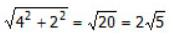Courses

# Test: Rectangular Solids- 2

## 20 Questions MCQ Test Quantitative Aptitude for GMAT | Test: Rectangular Solids- 2

Description
This mock test of Test: Rectangular Solids- 2 for GMAT helps you for every GMAT entrance exam. This contains 20 Multiple Choice Questions for GMAT Test: Rectangular Solids- 2 (mcq) to study with solutions a complete question bank. The solved questions answers in this Test: Rectangular Solids- 2 quiz give you a good mix of easy questions and tough questions. GMAT students definitely take this Test: Rectangular Solids- 2 exercise for a better result in the exam. You can find other Test: Rectangular Solids- 2 extra questions, long questions & short questions for GMAT on EduRev as well by searching above.
QUESTION: 1

### A toy is in the form of a right circular cone mounted on a hemisphere. If the height of the cone is twice the radius of the cone and the total height of the toy is 15 centimeters, what is the volume of the hemisphere in cubic centimeters? (The volume of a sphere is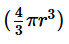, where r is the radius)

Solution:
• A toy is in the form of a right circular cone mounted on a hemisphere
• Total height of the toy = 15 centimeter
• Height of the cone = 2 * [radius of the cone]
• The volume of a sphere =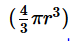, where r is the radius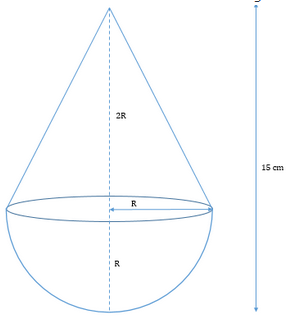To Find: Volume of hemisphere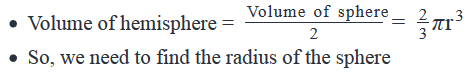Approach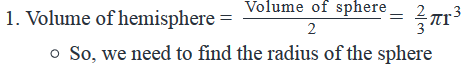• Let’s assume the radius of the hemisphere to be R.
• As the cone is mounted on the hemisphere, the

​​            Radius of the cone = Radius of the hemisphere = R

• Now, we are given the relation that Height of the cone(let’s assume to be H) = 2 * R

• Also, we know the total height of the cone = H + R = 15 centimeters

a.  From the above equation, we can find the value of R

Working Out

• Height of Cone(H) = 2R

• Height of Toy = H + R = 2R + R = 3R = 15, i.e. R = 5 centimeters

• Volume of hemisphere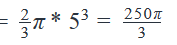QUESTION: 2

###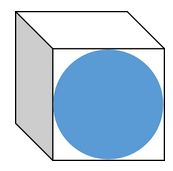The figure above shows a cube one of whose faces has been painted with a blue circle that touches each edge of the face. If all the other faces of the cube are also to be painted in a similar manner, how much surface area of the cube will be left unpainted? (1) The surface area of the cube is 1944 square units (2) The area of the blue circle is 81π square units

Solution:

Solution

Steps 1 & 2: Understand Question and Draw Inferences

Given:

• A cube – let the edge length of the cube be "a" units
• A circle that touches all edges of a cube – this means that:
• (Diameter of the circle) = (Edge length of the cube)
• Let the radius of the circle be r units. So, 2r = a
•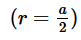To find:

• Total Area left unpainted in the cube
• Area left unpainted in 1 face of the cube = (Area of the face) – (Area of the blue circle)
•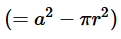Area left unpainted in all the 6 faces of the cube = 6(a2−πr2)

• This is our required value
• Remember that we already know that r=a2
• So, we only need to find the value of either r and a, and we will be able to find the value of the other quantity (using r=a/2
• , and thus find the value of  6(a2−πr2)

Step 3: Analyze Statement 1 independently

• Statement 1 says that ‘The surface area of the cube is 1944 square units’
• 6a2 = 1944
• From this equation, we will be able to get a unique value of a (ignoring the negative square root because the edge length of a cube cannot be negative)
• Therefore, we will be able to find the total unpainted area
• Hence, Statement 1 alone is sufficient to answer the question

Step 4: Analyze Statement 2 independently

• Statement 2 says that ‘The area of the blue circle is 81π square units’
• πr2=81π
• r2 = 81
• From this equation, we will be able to get a unique value of r (ignoring the negative square root because the radius of a circle cannot be negative)
• Therefore, we will be able to find the total unpainted area
• Hence, Statement 2 alone is sufficient to answer the question

Step 5: Analyze Both Statements Together (if needed)

Since we’ve already arrived at a unique answer in each of steps 3 and 4, this step is not required

Therefore, the correct answer is Option D.

QUESTION: 3

### A rectangular solid brick of iron is melted and shaped into a cube. If the areas of different sides of the brick were 24, 36 and 54 square units respectively, what is the surface area of the cube in square units?

Solution:

Given:

• Let the sides of the rectangular solid brick be L, B and H
• Let us assume that
• LB = 24
• BH = 36
• HL = 54
• Let the side length of the cube be “a” units

To find:

• Surface area of the cube

Approach and Working:

• We know that Surface area of the cube = 6a2
• So, to answer the question, we need to find the value of “a”.
• Since the cube is formed by melting the rectangular solid brick, we can conclude that:
• Volume of cube = Volume of brick
• So, if we can find the volume of brick, we can find the volume of cube, that is, a3, and hence the value of “a”.
• Finding the volume of the rectangular solid brick
• Let this volume be V
• So V = LBH
• Now, (LB)(BH)(HL) = 54*24*36
• So, (LBH)2 = (33*2)(3*23)(22*32)
• That is, V2 = 26*36
• V = 23*33
• Finding the value of a
• Volume of cube = Volume of brick
• So, a3 = 23*33
• Therefore, a = 2*3 = 6
• Finding the Surface Area of the cube
• Surface area of the cube = 6a2 = 6*62 = 63 = 216
• Looking at the answer choices, we see that the correct answer is Option C
QUESTION: 4

By looking at a rectangular box, a carpenter estimates that the length of the box is between 2 to 2.1 meters, inclusive, the breadth is between 1 to 1.1 meters, inclusive and the height is between 2 to 2.1 centimeters, inclusive. If the actual length, breadth and height of the box do indeed fall within the respective ranges estimated by the carpenter, which of the following is the closest to the maximum possible magnitude of the percentage error that the carpenter can make in calculating the volume of the rectangular box?

Solution:

Given:

• 2 meters ≤ Estimated Length of the rectangular box, LE ≤ 2.1 meters
• 1 meter ≤ Estimated Breadth of the rectangular box, BE ≤ 1.1 meters
• 2 centimeters ≤ Estimated Height of the rectangular box, HE ≤ 2.1 centimeters
• The actual L, B and H of the box fall within the respective estimated ranges

To find: Approx. value of the maximum % error in estimating the volume

Approach:

1. Error Percentage in Volume calculation =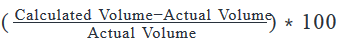• The magnitude of the error percentage will be maximum when the magnitude of the numerator is maximum and denominator is minimum.
• So, the case of Maximum possible error arises when:
1. the actual volume is in fact the least possible, given the ranges of LE, BE and HE AND
2. the carpenter calculates the volume using the maximum estimated values of LE, BE and HE

1. So we’ll calculate the least possible value of the actual volume and the greatest possible value of the calculated volume
• Note that in Volume calculations, we should convert the units of length and breadth into centimeters (to make the units of length, breadth and height uniform)

Working Out:

• Finding least possible value of the actual volume
• The least possible actual volume occurs when:
• L = 2 meters = 200 centimeters
• H = 2 centimeters
• B = 1 meter = 100 centimeters
• So, least possible actual volume = 200∗100∗2=40000 cm3

• Finding greatest possible value of the calculated volume
• The greatest possible calculated volume occurs when:
• Estimated length used for the calculation = 2.1 meters = 210 centimeters
• Estimated breadth used for the calculation = 1.1 meter = 110 centimeters
• Estimated height used for the calculation = 2.1 centimeters

So, greatest possible calculated volume = = 210*110*2.1 = =210∗11∗21 cm3

Finding the maximum % error

• So, Maximum error Percentage in Volume calculation =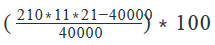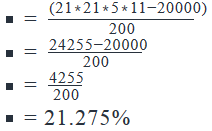Looking at the answer choices, we see that the answer choice which is closest to the value we obtained is Option E (22%) So, Option E is the correct answer

QUESTION: 5

A right circular cylinder having the radius of its base as 2 centimeters is filled with water upto a height of 2 centimeters. This water is then poured into an empty rectangular container the dimensions of whose base are 2π by 3 centimeters. If the volume of water in the rectangular container is increased by 50 percent by adding extra water, what is the final height, in centimeters, of the water level in centimeters in the rectangular container?

Solution:

Given

• Water is filled in a right circular cylinder
• Height of water in the cylinder = 2 centimeters
• Rectangular container with base dimensions as 2π by 3 centimeters
• Volume of the water in the container is increased by 50%

To Find:

• The height of the water in the container.

Approach and Working:

• The total volume of water in the rectangular container = Area of the base of the rectangular container * Height of the water in the rectangular container.
• As we know the dimensions of the base of the rectangular container we can calculate the area of its base.
• So, we need to find the total volume of water in the rectangular container to find the height of the water in the rectangular container
• Total Volume of water in the rectangular container = Initial volume of water + 50% of the initial volume of water.
• Now, we know that Initial volume of water = volume of water in the cylinder
• As we know the radius of the cylinder and the height of the water in the cylinder, we can calculate the volume of water in the cylinder.
• Area of the base of the rectangular container = 2π * 3 = 6π
• Volume of the rectangular container = Area of base * Height = 6π * H………(i)
• Total volume of water in the rectangular container = Initial volume of water + 50% of initial volume of water
• Initial volume of water = volume of water in the cylinder = π * 22 * 2
• Total volume of water in the rectangular container = 8π * 1.5 = 12π…………. (ii)
• Equating (i) and (ii), we get –
• 6π * H = 12π
• H = 2 centimeters
• Thus, the correct answer is Option D.
QUESTION: 6

What is the longest distance between two points in a rectangular solid if the area of its faces is 50, 75 and150 square units respectively?

Solution:

Given

• Area of faces of a rectangular solid:
• Face -1 = 50 square units
• Face-2 = 75 square units
• Face-3 = 150 square units

To Find: Length of the longest distance between two points inside the solid.

Approach

1.     Length of the longest distance between 2 points inside the solid = (length of the space diagonal) =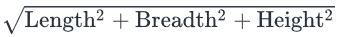So, we need to find the dimensions of the rectangular solid

2. Let’s assume the length, breadth and height of the rectangular solid be l, b and h respectively.
So, we have

1. l * b = 50……..(1)
2. b * h = 75 ……(2)
3. l * h = 150……(3)
3. As we have 3 equations and 3 unknowns we will solve the above equations to find the value of l, b and h.

Working Out

1. Multiplying (1), (2) and (3), we have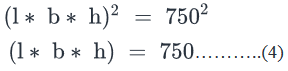1. Substituting equation (1) in (4), we have, h = 15

2. Substituting equation (2) in (4), we have, l = 10

3. Substituting equation (3) in (4), we have, b = 5

2. Length of space diagonal =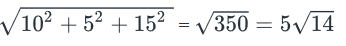QUESTION: 7

Water is taken out of a cylindrical bucket by filling a cylindrical mug to the brim. How many mugs of water does the bucket contain?

1. The height of the bucket is three times the height of the mug and the radius of the bucket is twice the diameter of the mug
2. The bucket is filled to 70 percent of its capacity
Solution:

Steps 1 & 2: Understand Question and Draw Inferences

Given:

• Water in a cylindrical bucket:
• Let the radius of the bucket be R and the height of water in the bucket be H
• So, the volume of the water in the bucket, V = πR2H
• Cylindrical Mug:
• Let the radius of the mug be r and the height of mug be h
• So, the volume of the mug, v = πr2h

To find: Number of mugs of water in the bucket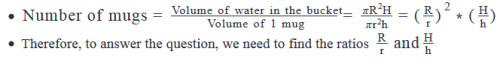Step 3: Analyze Statement 1 independently

Statement 1 says that ‘The height of the bucket is three times the height of the mug and the radius of the bucket is twice the diameter of the mug’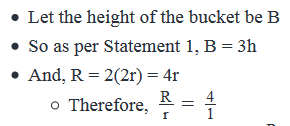• Statement 1 gives us the ratio R/r but doesn’t give us the other required ratio, H/h
• Therefore, Statement 1 alone is not sufficient to answer the question

Step 4: Analyze Statement 2 independently

Statement 2 says that ‘The bucket is filled to 70 percent of its capacity’

• This means, (Volume of water in the bucket) = 70% of (Volume of Bucket)
• We have assumed the height of water in the bucket to be H. Let’s now assume the height of the bucket to be B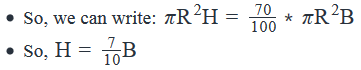• Note that this statement has given us no information about the required ratios, RrandHh
• So, Statement 2 is not sufficient

Step 5: Analyze Both Statements Together (if needed)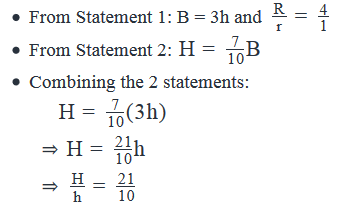• Thus, we now know the values of both the required ratios, R/r and H/h
• So, the 2 statements together are sufficient to answer the question

QUESTION: 8

A cube of side length x centimeters is placed on a floor. A cube of side length x/2 centimeters is placed at the center of the top of the cube of side length x centimeters. What fraction of the total surface area of the cubes is visible?

Solution:

Given

• Cube of side length x centimeters.
• Let’s call this cube B
• Cube of side length x2
•  place on top of cube B.
• Let’s call this cube as cube A

To Find: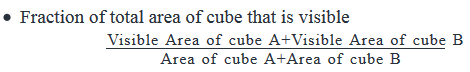Approach

• Since we have expressed the sides of both the cubes in terms of x, we can find the area of cube A and area of cube B in terms of x
• Let’s find out the visible areas of cubes A and B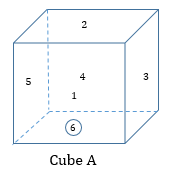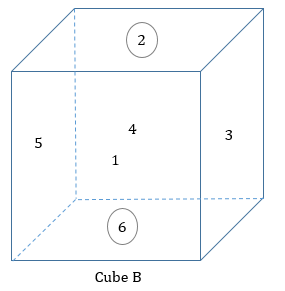• Visible area of cube A
• For cube A, the area that is not visible is the face placed on the top of cube B, i.e. face 6
• All the other faces of cube A are completely visible
• So, visible areas of Cube A = Area of 5 faces of cube A

• Visible area of cube B
• For cube B, the area that is completely not visible is the face placed on the ground, i.e. face 6
• Also, the top of the cube B is partially visible because cube A is placed on the top of cube A. Hence, face 2 is partially visible
• The area of face 2 that is visible = Total area of face 2 – Area of face 6 of cube A
• So, visible areas of Cube B = 4 faces of cube B + partial area of face 2
• Also, we know that area of one face of cube B = x20

Working Out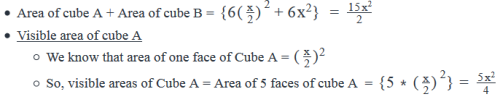Visible area of cube B
a. We know that area of one face of cube B = x2
b. So, visible areas of Cube A =Area of 4 faces of cube B +partial area of face 2 =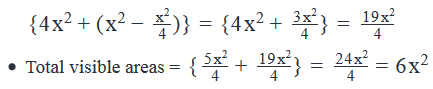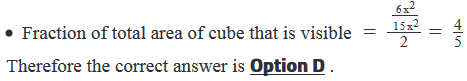QUESTION: 9

If a cube of side length 24 units is cut into 512 smaller cubes of equal dimensions, then what is the ratio of the combined surface area of the smaller cubes and the surface area of the original cube?

Solution:

Given:

• Edge Length of bigger cube = 24 units
• Let the edge length of each smaller cube be a units
• Number of smaller cubes = 512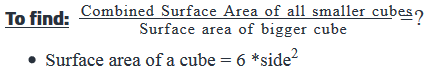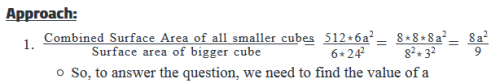2. We’re given the number of smaller cubes. As the smaller cubes have been formed from the larger cubes,

(Volume of bigger cube) = (Combined Volume of 512 smaller cubes). So, we can find a from the above equation.

Working Out: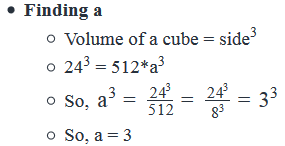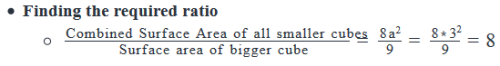Looking at the answer choices, we see that the correct answer is Option C

QUESTION: 10

A hall is in the form of a rectangular solid with its each side length equal to 12 feet. If the floor and one wall of the hall are to be covered with colored tiles measuring 2 feet by 2 feet, how many such tiles are required?

Solution:

Given

• Length of each side of the rectangular solid = 12 feet
• Floor and one wall are to be covered by rectangular tiles
• Dimension of tiles = 2 by 2 feet

To Find: Number of tiles required?

Approach

1. Number of tiles required =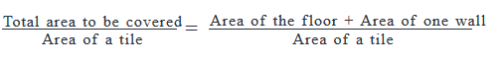1. As we know the side length of the hall, we can find the area of floor = area of one wall = (side)2
2. Also, as we know the dimensions of the tiles, we can find its area = (2*2) square feet

Working Out

1. Area of floor = Area of one wall = 122=144
2.  square feet
1. Area of floor + Area of one wall = 144 + 144 = 288 square feet

3. Area of a tile = 2 * 2 = 4 square feet

4. Number of tiles required = 288/4=72

QUESTION: 11

The volume of a sphere with radius r is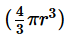and the surface area is 4πr2. If a solid spherical ball of iron with radius r centimetres is cut into two equal hemispheres, what is the ratio, in terms of r, of the volume and the surface area of each hemisphere?

Solution: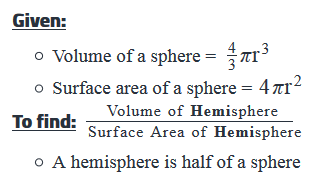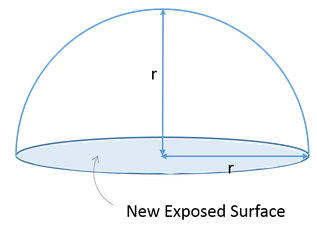Approach:

1. To find the required ratio, we need to know the volume and the surface area of the hemisphere.
2. Volume of hemisphere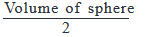3. Surface area of hemisphere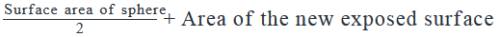Note that the new exposed surface is a circle of radius r units

Working Out: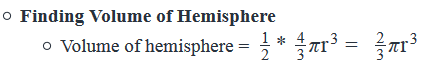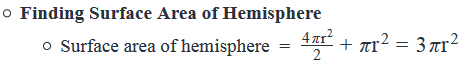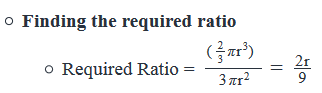So, the correct answer is Option A

QUESTION: 12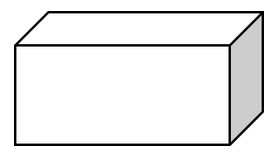In the rectangular solid above, if each dimension of the rectangular solid is an integer greater than 1 and the area of two sides of the solid is 14 and 18 respectively, what is the volume of the solid?

Solution:

Given:

• Let the dimensions of the rectangular solid be L, B, H.
• L, B and H are integers
• L > 1, B>1 and H>1
• Let L*B = 14
• And, let B*H = 18

To find: Volume of the rectangular solid

Approach:

1. Volume of the rectangular solid = L*B*H
• Since we’re given the values of L*B and B*H, we can write L∗B∗H=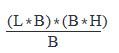• So, to find the volume, we need to find the value of B
1. Integer B is the common divisor of L*B and B*H and is greater than 1. Since we’re given the values of L*B and B*H, we can find the common divisors of these 2 values.
• If we get only one common divisor (of L*B and B*H) greater than 1, then we’ll be able to find a unique value of B, and hence of the volume
• If we get multiple common divisors greater than 1, then we’ll get multiple values of B, and hence of the volume. In this case, the answer will become ‘(A unique value) cannot be determined’

Working Out:

• Finding the common divisor(s) of L*B and B*H
• L*B = 14 = 2*7
• B*H = 18 = 2*32
• So, the common divisors of L*B and B*H = {1, 2}
• The only common divisor greater than 1 is 2
• So, B = 2

• Finding the volume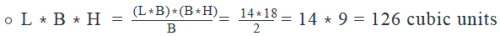Looking at the answer choices, we see that the correct answer is Option B

QUESTION: 13

The space diagonal of cube A is more than 2√6 units longer than the space diagonal of cube B and the surface area of cube A is 192 square units greater than the surface area of cube B. If the lengths of both cubes are integers, by how many cubic units is the volume of cube A greater than the volume of cube B?

Solution:

Given:

• Let the edge length of cube A be A units and of cube B be B units
• A and B are (positive) integers
• (Space Diagonal of Cube A) > (Space Diagonal of Cube B) + 2√6
1. √3A > √3B + 2√6
• (Surface Area of Cube A) = (Surface Area of Cube B) + 192
1. 6A2 = 6B2 + 192

To find: (Volume of Cube A) – (Volume of Cube B) = ?

Approach:

1. (Volume of Cube A) – (Volume of Cube B) = A3 – B3
• So, to answer the question, we need to find the value of A and B
2. We’re given that 6A2 = 6B2 + 192
• That is, A2 – B2 = 32
• We’ll find the possible sets of A and B that satisfy this equation (remember that A and B are positive integers)
3. Then, on the sets of values obtained above, we’ll apply the constraint that √3A > √3B + 2√6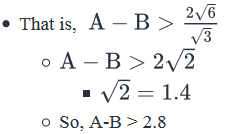• Using this constraint, we’ll eliminate all the sets of values that don’t satisfy this constraint. If we’re left with only one possible set of values of A and B, then we’ll be able to find a unique answer to the question. Else, the answer will probably be Option E.

Working Out:

• Solving A2 – B2 = 32 and applying the constraint A-B > 2.8
• (A+B)(A-B) = 32
• Since A and B are integers, A + B and A – B will be integers as well.
• So, to solve this equation, we should express 32 as a product of integers. This can be done in multiple ways:
• Case 1: 32 = 32*1
• So, (A+B)(A-B) = 32*1
• Now, A is clearly bigger than B.
• And since both A and B are positive, A+ B will be bigger than A- B
• So, A+B = 32
• A – B = 1
• But this value of A – B violates the constraint that A – B > 2.8. So, Case 1 is rejected.
• Case 2: 32 = 16*2
• So, (A+B)(A-B) = 16*2
• Therefore, A+B = 16
• A – B = 2
• But this value of A – B violates the constraint that A – B > 2.8. So, Case 2 is rejected.
• Case 3: 32 = 8*4
• So, (A+B)(A-B) = 8*4
• So, A + B = 8 . . . (1)
• A – B = 4 . . . (2)
• This value of A – B does satisfy the constraint
A – B > 2.8. So, Case 3 is accepted.
• 2A = 12
• So, A = 6
• From (1), B = 2

• Thus, we’ve found that A = 6 and B = 2

• Volume of Cube A – Volume of Cube B = A3 – B3 = 63 – 23 = 216 – 8 = 208 cubic units

Looking at the answer choices, we see that the correct answer is Option B

QUESTION: 14

A right circular cylinder of height 1 meter and radius 9√2 centimeters is to be used to store cubes of side length 3 cm each. If in each layer of cubes stored in the cylinder, the cubes are arranged such that the top view of the layer is a square, what is the maximum number of cubes that can be stored in the cylinder? (1 meter = 100 centimeters)

Solution:

Given:

• Right circular cylinder
• Height, say H = 1 meter = 100 cm (converting the height into centimeters since all other lengths are given in centimeters)
• Radius, say R = 9√2 cm
• Cubes
• Edge Length = 3 cm
• The cubes are arranged in layers inside the cylinder such that each layer of cubes forms a square on top-view of the cylinder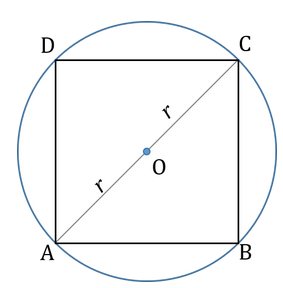To find: The maximum number of cubes that can be stored in the cylinder

Approach:

1. The maximum number of cubes will be stored when:
• The number of layers of cubes inside the cylinder is the maximum possible
• And each layer contains the maximum possible number of cubes
2. Maximum number of layers will be equal to the quotient that is obtained when the Height of the cylinder is divided by the height of the cube
• For example, if the height of the cylinder is 10 units and
• the height of the cube is 2 units, then 5 layers of cube are possible
• But if the height of the cube is 3 units, then only 3 layers of cube will be possible and there will be empty space left of 1-unit height in the cylinder
3. The maximum possible number of cubes will result when the square top-view of each layer looks as much as possible as square ABCD in the diagram above.
• Note that ABCD is the biggest square that can be drawn inside a circle of radius r. The diagonal of this square is equal to the diameter of the circle.

Working Out:

• Finding the maximum possible number of layers
• Total height of the cylinder, H = 1m = 100 cm
• The height of each layer = 3 cm (edge length of the cubes)
• The quotient obtained when 100 is divided by 3 is 33.
• Therefore, the maximum possible number of layers is 33.

• Finding the maximum possible number of cubes per layer
• Diagonal of ABCD = Diameter of circle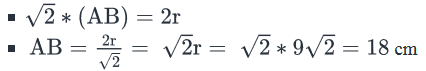• Note that the side length of ABCD, 18 cm, is a multiple of the edge length of each cube (3 cm)
• This means that when you start lining cubes along the side AB of the square, no extra space (meaning, space that is not occupied by cubes) will be left on AB. The side AB of the square will be completely lined by 6 cubes. Similarly, each side of the square ABCD will be lined by 6 cubes.
• Total number of squares that will fit into square ABCD =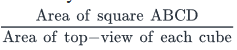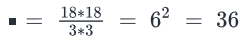• Thus, in the most tightly packed arrangement, each layer of cubes contains 36 cubes.
• So, total number of cubes in most tightly packed arrangement = (Maximum possible number of cubes in each layer)*(Maximum possible number of layers)
• = 36*33
• = 1188

• Looking at the answer choices, we see that the correct answer is Option D
QUESTION: 15

A right circular cylinder is cut parallel to its base into two halves. By what percentage is the combined total surface area of the smaller cylinders greater than the total surface area of the original cylinder?

(1)  The sum of the radius and the height of the original cylinder is 3 times the radius of the original cylinder.

(2)  If the original cylinder is melted and a part of the molten material is used to form into a sphere with the same radius as the original cylinder, the volume of the sphere thus formed will be 33 percent less than the volume of the original cylinder. (The volume of a sphere is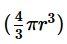, where r is the radius)

Solution:

Steps 1 & 2: Understand Question and Draw Inferences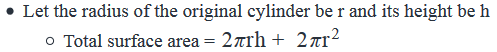Radius of each of the smaller cylinder = r  and height of each of the smaller cylinder = h/2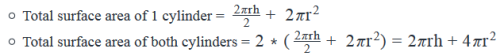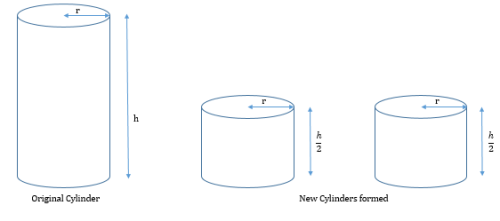To Find: Percentage increase in total surface area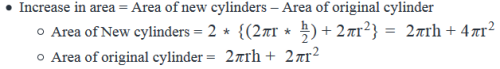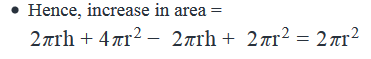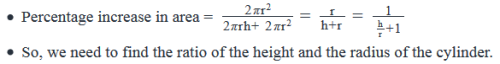Step 3: Analyze Statement 1 independently

(1)  The sum of the radius and the height of the original cylinder is 3 times the radius of the original cylinder.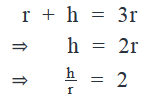Step 4: Analyze Statement 2 independently

(2)  If the original cylinder is melted and a part of the molten material is used to form a sphere with the same radius as the original cylinder, the volume of the sphere thus formed will be 33 percent less than the volume of the original cylinder. (The volume of a sphere is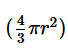, where r is the radius)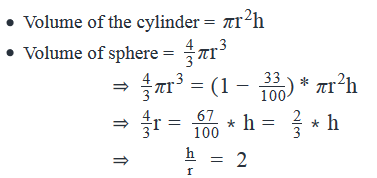Step 5: Analyze Both Statements Together (if needed)

As we have a unique answer from steps 3 and 4, this step is not required.

QUESTION: 16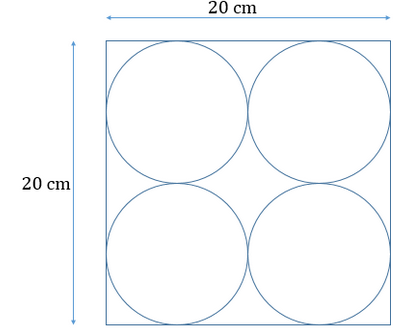In a rectangular box of dimensions 20 centimetres by 20 centimetres by 100 centimetres, identical spherical balls are arranged in layers such that each layer has exactly 4 balls. The top view of this arrangement is shown in the figure above. What is the approximate percentage of space left empty inside the box, if the box contains the maximum possible number of layers of such spherical balls? (The volume of a sphere is, where r is the radius of the sphere)

Solution:

Given

• Dimension of rectangular box = 20 by 20 by 100 centimeters
• Number of spherical balls in each layer = 4
• Volume of a sphere =• , where r is the radius of the sphere

To Find: Approximate percentage of empty space left inside the box?

Approach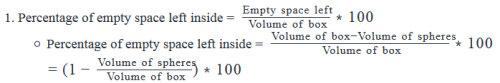2. Volume of box

a. As we know the dimensions of the box, we can find the volume of the box

3. Volume of total spheres in the box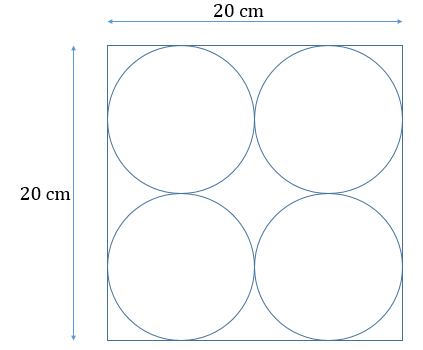• As all the spheres in the box are identical, they will have the same volume. So, we can write
1. (Volume of total spheres in the box) = (Volume of 1 sphere * Number of spheres in the box).
• As we can see that the {length of the box} = [2 * diameter of the sphere] = [4 * radius of the sphere]
1. Since, we know the length of the box, we can find the radius of a spherical ball
2. Thus, we can find the volume of a sphere, however we also need to find the number of spheres in the box

• Number of spheres in the box
1. Each layer of the spherical balls will have a height equal to the diameter of a spherical ball.
2. Since the balls in a layer are aligned along the dimensions 20 by 20, the number of layers of spherical balls will depend on the height of the box, i.e. 100 centimeters
3.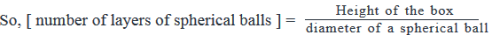1. We can write this equation because we are given that the number of layers in the box is the maximum possible. Had this relation not been given we could not have used the height of the box in the numerator of the equation.​

Working Out

1. [ Volume of box = 20 * 20 * 100 (cm)3]
2. Volume of spheres in the box
1. Let’s assume the radius of a spherical ball to be r centimeters
1. So, 4r = 20 i.e. r = 5 centimeters
2. Thus, volume of a sphere =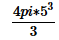3. ​​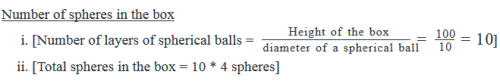4. [ Total volume of spheres in the box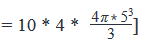3. . Percentage of empty space left inside =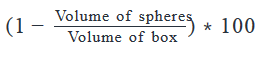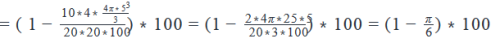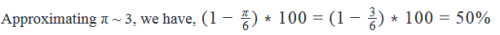QUESTION: 17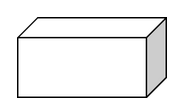In the rectangular solid above, if each dimension of the solid is an integer, what is the volume of the solid?

(1) The area of two sides of the rectangular solid is 4 and 20 respectively

(2) The area of two sides of the rectangular solid is 4 and 5 respectively.

Solution:

Solution

Steps 1 & 2: Understand Question and Draw Inferences

Let the length, breadth, and height of the rectangular solid be L, B and H respectively.

We are given that L, B, H are integers.

We need to find the volume of the solid, that is, the value of the product LBH.

Step 3: Analyze Statement 1 independently

• Statement 1 states that "The area of two sides of the rectangular solid is 4 and 20 respectively
• Let the two sides whose area is 4 be B and L, and the two sides whose area is 20 be B and H.
• So, we can write: B*L = 4
• In how many ways can 4 be expressed as a product of 2 numbers?
• We can write 4 = 1*4 or 2*2
• This means (B,L) are either (1,4) or (2,2)

• Let’s now analyze the second piece of information given in St. 1
• B*H = 20 = 1*20 = 2*10 = 4*5
• This means (B,H) are either (1,20) or (2,10) or (4,5)

• Note that the pairs of sides (B,L) and (B,H) share one dimension – B.
• Applying this constraint on the possible values of (B,L) and (B,H) deduced above, we get: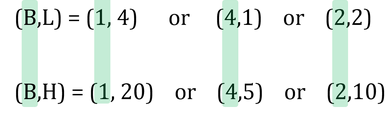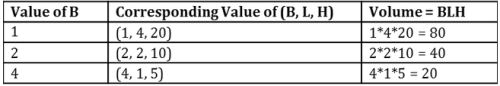• Since we’ve not been able to determine a unique value of the volume, Statement 1 alone is not sufficient.

Step 4: Analyze Statement 2 independently

Statement 2 states that: The area of two sides of the rectangular solid is 4 and 5 respectively.

• Let the two sides whose area is 4 be B and L, and the two sides whose area is 5 be L and H.
• So, we can write: L*B = 4 = 1*4 = 2*2
• This means (L,B) is either (1,4) or (2,2)
• Also, L*H = 5 = 1*5
• Since 5 is a prime number, it can only be expressed as a product of 1 and 5 itself.
• This means (L, H) = (1,5)
• Now, the pairs of sides (L,B) and (L,H) share one dimension – L
• Applying this constraint on the possible values of (L,B) and (H,L) deduced above, we get:
• L = 1, H = 5 and B = 4
• Therefore, LBH = 1*4*5 = 20
• Since Statement 2 leads us to a unique value of the volume of the solid, it is sufficient.

Step 5: Analyze Both Statements Together (if needed)

Since we have a unique answer from step 4, this step is not required.

Hence the correct answer is Option B

QUESTION: 18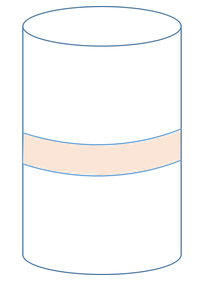A paint can is in the shape of a right circular cylinder. A rectangular paper label of area 12 square units is pasted on the can as shown in the figure.If the label can be wrapped around the can only once with no extra paper hanging out, what is the curved surface area of the paint can?

(1) The center of the paper label is at a distance of 5 units each from the top and the bottom of the can

(2) The height of the can is five times the height of the paper label.

Solution:

Steps 1 & 2: Understand Question and Draw Inferences

Given:

• A right circular cylinder
• Let radius = R units
• Let height = H units
• A paper label of area 12 square units
• Let height of paper label = h units
• Length of the paper label = Circumference of the top-view circle of the cylinder = 2πR
• So, area of paper label = 2πRh=12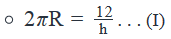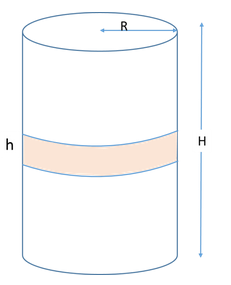To find: The curved surface area of the paint can

• Curved Surface Area = A=2πRH . . . (II)
• Substituting (I) in (II):
• A=12H/h  . . . (III)

Thus, in order to find the required area, we need to find the ratio H/h

Step 3: Analyze Statement 1 independently

(1) The center of the paper label is at a distance of 5 units each from the top and the bottom of the can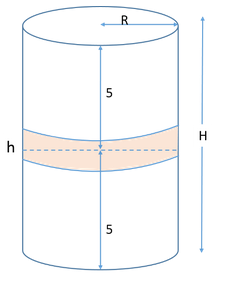• H = 5 + 5 = 10
• But we don’t know the value of h yet.

So, statement 1 is not sufficient.

Step 4: Analyze Statement 2 independently

(2) The height of the can is five times the height of the paper label.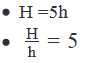Since we now know the value of , we can find a unique value of A.

Sufficient.

Step 5: Analyze Both Statements Together (if needed)

Since we’ve already arrived at a unique answer in Step 4, this step is not required

QUESTION: 19

If x units are added to the length of the radius of a circle, what is the number of units by which the circumference of the circle is increased?

Solution:

Let the radius of the circle be 'r' units.
The circumference of the circle will therefore be 2πr units.

If the radius is increased by 'x' units, the new radius will be (r + x) units.

The new circumference will be
2π(r+x) = 2πr +2πx
Or the circumference increases by 2πx units.

QUESTION: 20

ABCD has area equal to 28. BC is parallel to AD. BA is perpendicular to AD. If BC is 6 and AD is 8, then what is CD?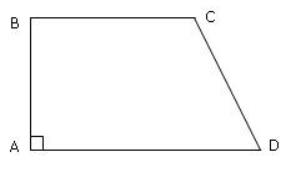Solution:

The given shape is a trapezium.
Area of a trapezium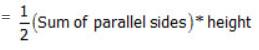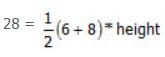Height = 4.

BA is perpendicular to BC and AD.

So, drop a line parallel to BA from C to meet AD at E.
CED is a right triangle with side CE measuring 4 and ED measuring 2 units.

Hence, CD, the measure of the hypotenuse  =